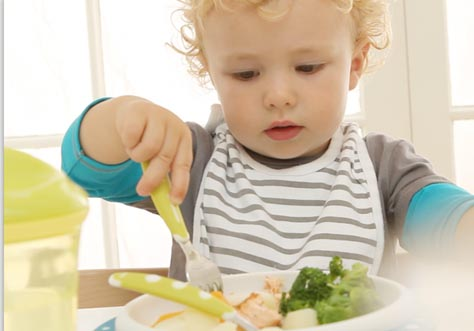It seems you are using an unsupported browser. Please update your browser to Chrome or Firefox for the complete NUK website experience.#### 這些適合這一階段的生活

1. <
2. <
3. <
4. <
5. <
6. <
7. <
8. <
9. <
10. <
11. <
12. <
13. <
14. <
15.Premium Choice 300mL 寬口PPSU奶瓶/矽膠奶嘴連手柄 - 獅子/海獅
\$139.00
Inkl. MwSt., zzgl. Versand
16. <
17. <
18. <
19. <
20. <
21. <
22. <
23. <
24. <
25. <
26. <
27. <
28. <
29. <
30. <
31. <
32. <
33. <
34. <
35. <
36. <
37. <
38. <
39. <
40. <
41. <
42. <
43. <
44. <
45. <
46. <
47. <
48. <
49. <
50. <
51. <
52. <
53. <
54. <
55. <
56. <
57. <
58. <
59. <
60. <
61. <
62. <
63. <
64. <
65. <
66. <
67. <
68. <
69. <
70. <
71. <
72. <
73. <
74. <
75. <
76. <
77. <
78. <
79. <
80. <